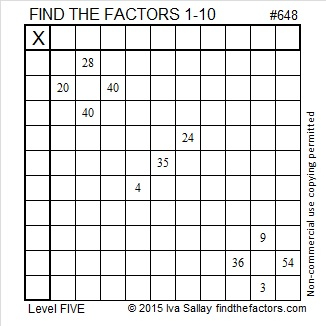# 648 and Level 5

648 is the sum of consecutive prime numbers 317 and 331.

The sixth root of 648 begins with 2.941682753. Notice all the digits from 1 to 9 appear somewhere in those nine decimal places. OEIS.org states that 648 is the smallest number that can make that claim.

From Archimedes-lab.org I learned some powerful facts about the number 648:

• 16² – 17² + 18² – 19² + 20² – 21² +22² – 23² + 24² – 25² + 26² – 27² + 28² – 29² + 30² – 31² + 32² = 648
• 48² – 47² + 46² – 45² + 44² – 43² +42² – 41² + 40² – 39² + 38² – 37² + 36² – 35² + 34² – 33² = 648
• (1^2)(2^3)(3^4) = 648
• 18² + 18²  = 648
• (6^3) + (6^3) + (6^3) =648Print the puzzles or type the solution on this excel file: 10 Factors 2015-10-12

—————————————————————————————————

• 648 is a composite number.
• Prime factorization: 648 = 2 x 2 x 2 x 3 x 3 x 3 x 3, which can be written 648 = (2^3) x (3^4)
• The exponents in the prime factorization are 3 and 4. Adding one to each and multiplying we get (3 + 1)(4 + 1) = 4 x 5 = 20. Therefore 648 has exactly 20 factors.
• Factors of 648: 1, 2, 3, 4, 6, 8, 9, 12, 18, 24, 27, 36, 54, 72, 81, 108, 162, 216, 324, 648
• Factor pairs: 648 = 1 x 648, 2 x 324, 3 x 216, 4 x 162, 6 x 108, 8 x 81, 9 x 72, 12 x 54, 18 x 36, or 24 x 27
• Taking the factor pair with the largest square number factor, we get √648 = (√324)(√2) = 18√2 ≈ 25.455844122…—————————————————————————————————This site uses Akismet to reduce spam. Learn how your comment data is processed.